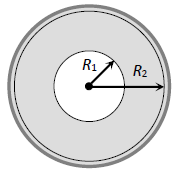Gravitational field intensity
Question

# A sphere of mass M and radius R2 has a concentric cavity of radius R1 as shown in figure. The force F exerted by the sphere on a particle of mass m located at a distance r from the centre of sphere varies as $\left(0\le \mathrm{r}\le \mathrm{\infty }\right)$Moderate
Solution

## because intensity is zero inside the cavity.F increase when ${\mathrm{R}}_{1}\le \mathrm{r}\le {\mathrm{R}}_{2}$

Get Instant Solutions